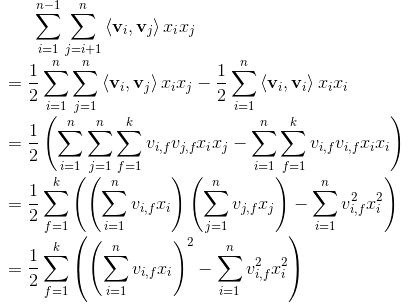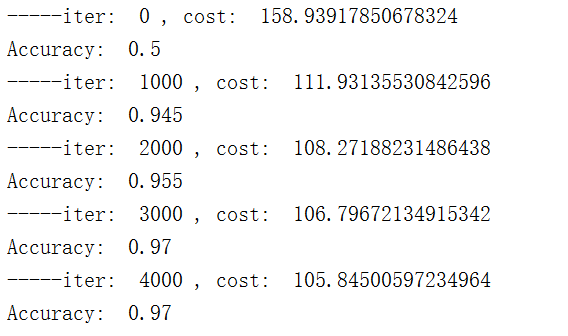#Factorization Machine

0
0
01. 云栖社区>
2. 博客>
3. 正文

# Factorization Machine---因子分解机

### ①target function的推导

logistics regression algorithm model中使用的是特征的线性组合，最终得到的分割平面属于线性模型，但是线性模型就只能处理线性问题，所以对于非线性的问题就有点难处理了，对于这些复杂问题一般是两种解决方法①对数据本身进行处理，比如进行特征转换，和函数高维扩展等等。②对算法模型本身进行扩展，比如对linear regression加上正则化惩罚项进行改进得到lasso regression或者是ridge regression。
Factorization Machine就是一种对logistics regression的一种改进，线性的部分权值组合是不变的，在后面增加了非线性的交叉项。
target function：表示的是系数矩阵V的第i维向量，，k的大小称为是度，度越大代表分解出来的特征就越多。对于每一个特征都会对应有一个维的向量。前两部分是传统的线性模型，后一个部分就是将脸刚刚互不相同的特征分量之间的相互关系考虑进来了。也就是不同特征之间的吸引程度。

### 优化求解target function对于原始的target function计算复杂度是，采用公式的公式。于是化简一波：FM可以解决的问题主要是四种：
1.回归问题：这时候的error function：2.二分类问题：3.排序问题。
4,推荐系统。### Factorization Machine的优点

①对于一些很稀疏的数据集也可以进行参数的预测，而SVM是不行的。
②FM有线性的复杂性，可以直接在原始数据进行预测，而不需要再做核函数或者特征转换，对于SVM，是要基于对支持向量的优化的。
③FMs是一种通用的预测器，可用于任何实值特征向量。相比之下。其他最先进的因数分解模型只在非常有限的输入数据上工作。通过定义输入数据的特征向量，FMs可以模拟最先进的模型，如偏置MF、SVD++、PITF或FPMC。

### 代码实现

import numpy as np
import pandas as pd
import matplotlib.pyplot as plt

def Accuracy(preiction, classlabel):
score = 0
for i in range(len(preiction)):
if preiction[i] > 0.5:
preiction[i] = 1
else:
preiction[i] = -1
if preiction[i] == classlabel[i]:
score += 1
print('Accuracy: ', score/len(preiction))

def initialize(n, k):
v = np.mat(np.zeros((n, k)))
for i in range(n):
for j in range(k):
v[i, j] = np.random.normal(0, 0.2)
return v

def sigmoid(inx):
return 1.0/(1+np.exp(-inx))

def getCost(predict, classLabels):
m = len(predict)
error = 0.0
for i in range(m):
error -= np.log(sigmoid(predict[i]*classLabels[i]))
return error

def getPrediction(dataMatrix, w0, w, v):
m = np.shape(dataMatrix)
result = []
for x in range(m):
inter_1 = dataMatrix[x] * v
inter_2 = np.multiply(dataMatrix[x], dataMatrix[x]) * np.multiply(v, v)
interaction = np.sum(np.multiply(inter_1, inter_1) - inter_2) / 2
p = w0 + dataMatrix[x] * w + interaction
pre = sigmoid(p[0, 0])
result.append(pre)
return result

def stocGradAscent(dataMatrix, classLabels, k, max_iter, alpha):
#initialize parameters
m, n = np.shape(dataMatrix)
w = np.zeros((n, 1))
w0 = 0
v = initialize(n, k)
#training
for it in range(max_iter):
for x in range(m):
inter_1 = dataMatrix[x] * v
inter_2 = np.multiply(dataMatrix[x], dataMatrix[x])*np.multiply(v, v)
interaction = np.sum(np.multiply(inter_1, inter_1) - inter_2)/2
p = w0 + dataMatrix[x]*w + interaction
loss = sigmoid(classLabels[x] * p[0, 0]) - 1
w0 = w0 - alpha*loss*classLabels[x]
for i in range(n):
if dataMatrix[x, i] != 0:
w[i, 0] = w[i, 0] - alpha*loss*classLabels[x]*dataMatrix[x, i]
for j in range(k):
v[i, j] = v[i, j] - alpha*loss*classLabels[x]*(dataMatrix[x, i]*inter_1[0, j]-v[i, j]*dataMatrix[x, i]*dataMatrix[x, i])
if it % 1000 == 0:
print('-----iter: ', it, ', cost: ', getCost(getPrediction(np.mat(dataMatrix), w0, w, v), classLabels))
Accuracy(getPrediction(np.mat(dataMatrix), w0, w, v), classLabels)

if __name__ == '__main__':



import numpy as np
import pandas as pd
import matplotlib.pyplot as plt

df = pd.read_csv(filename, sep='    ', names=['1', '2', 'target'])
data = []
target = []
for i in range(len(df)):
d = df.iloc[i]
ds = [d['1'], d['2']]
t = int(d['target'])
if t == 0:
t = -1
data.append(ds)
target.append(t)
return np.mat(data), np.mat(target).tolist()

if __name__ == '__main__':
print(dataMatrix)
print(target)https://github.com/GreenArrow2017/MachineLearning/tree/master/MachineLearning/Factorization%20Machine

+ 关注